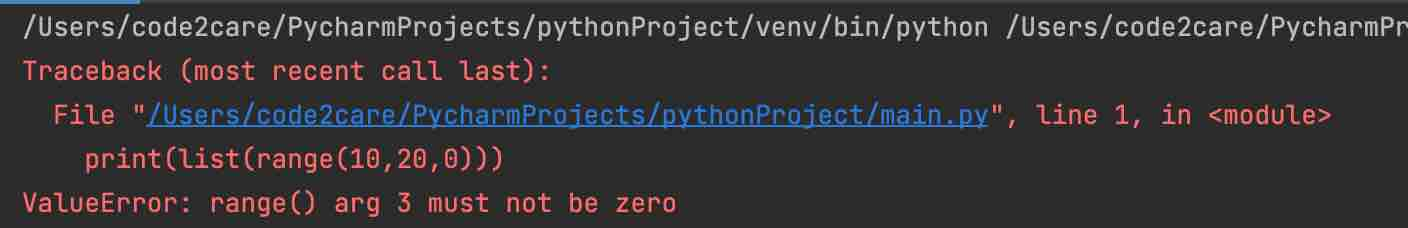# Python range() function examples

In Python range() is an inbuilt function, it represents an immutable sequence of numbers, you may mostly use it for looping a specific number of times in for loops.

Syntax:
``````range(stop)
range(start,stop)
range(start,stop,step)``````
• start: It is a value of the start parameter, zero by default. Optional
• stop: It is an value defining where to stop: Mandatory
• step: It is an value for inclementation. Optional
Rules for range() function
• The arguments to range function i.e. stop, start and stop can only byte int values.
• Only stop argument is mandatory.
• If you use syntax: range(stop), the start value is 0.
• If you use syntax: range(start,stop), the step value for increment is 1.
• If you use syntax: range(start,stop,step), the step value for cannot be 0

## Range with one constructor argument: range(stop)

Example 1:
``````no = range(5)
for i in no:
print("Hello Python!")``````
Output:

Hello Python!
Hello Python!
Hello Python!
Hello Python!
Hello Python!

Example 2:
``print(list(range(10)))``
Output:

[0, 1, 2, 3, 4, 5, 6, 7, 8, 9]

## Range with two constructor argument: range(start, stop)

Example 3:
``````for i in range(1, 3):
print("Hello Python!")``````
Output:

Hello Python!
Hello Python!

Example 4:
``print(list(range(1, 11)))``
Output:

[1, 2, 3, 4, 5, 6, 7, 8, 9, 10]

## Range with three constructor argument: range(start, stop, step)

Example 5:
``````for i in range(1, 11, 1):
print(i*2)``````
Output:

2
4
6
8
10
12
14
16
18
20

Example 6:
``print(list(range(0, 40, 5)))``
Output:

[0, 5, 10, 15, 20, 25, 30, 35]

Note: if you provide step as zero, you will get ValueError error,

``````Traceback (most recent call last):
File "/Users/code2care/PycharmProjects/pythonProject/main.py", line 1, in &t;module>
print(list(range(10,20,0)))
ValueError: range() arg 3 must not be zero``````Python documentation for range: https://docs.python.org/3/library/stdtypes.html?highlight=range#range

-

Have Questions? Post them here!